# sklearn.svm.SVC¶

class sklearn.svm.SVC(*, C=1.0, kernel='rbf', degree=3, gamma='scale', coef0=0.0, shrinking=True, probability=False, tol=0.001, cache_size=200, class_weight=None, verbose=False, max_iter=-1, decision_function_shape='ovr', break_ties=False, random_state=None)[source]

C-Support Vector Classification.

The implementation is based on libsvm. The fit time scales at least quadratically with the number of samples and may be impractical beyond tens of thousands of samples. For large datasets consider using LinearSVC or SGDClassifier instead, possibly after a Nystroem transformer.

The multiclass support is handled according to a one-vs-one scheme.

For details on the precise mathematical formulation of the provided kernel functions and how gamma, coef0 and degree affect each other, see the corresponding section in the narrative documentation: Kernel functions.

Read more in the User Guide.

Parameters:
Cfloat, default=1.0

Regularization parameter. The strength of the regularization is inversely proportional to C. Must be strictly positive. The penalty is a squared l2 penalty.

kernel{‘linear’, ‘poly’, ‘rbf’, ‘sigmoid’, ‘precomputed’} or callable, default=’rbf’

Specifies the kernel type to be used in the algorithm. If none is given, ‘rbf’ will be used. If a callable is given it is used to pre-compute the kernel matrix from data matrices; that matrix should be an array of shape (n_samples, n_samples).

degreeint, default=3

Degree of the polynomial kernel function (‘poly’). Ignored by all other kernels.

gamma{‘scale’, ‘auto’} or float, default=’scale’

Kernel coefficient for ‘rbf’, ‘poly’ and ‘sigmoid’.

• if gamma='scale' (default) is passed then it uses 1 / (n_features * X.var()) as value of gamma,

• if ‘auto’, uses 1 / n_features.

Changed in version 0.22: The default value of gamma changed from ‘auto’ to ‘scale’.

coef0float, default=0.0

Independent term in kernel function. It is only significant in ‘poly’ and ‘sigmoid’.

shrinkingbool, default=True

Whether to use the shrinking heuristic. See the User Guide.

probabilitybool, default=False

Whether to enable probability estimates. This must be enabled prior to calling fit, will slow down that method as it internally uses 5-fold cross-validation, and predict_proba may be inconsistent with predict. Read more in the User Guide.

tolfloat, default=1e-3

Tolerance for stopping criterion.

cache_sizefloat, default=200

Specify the size of the kernel cache (in MB).

class_weightdict or ‘balanced’, default=None

Set the parameter C of class i to class_weight[i]*C for SVC. If not given, all classes are supposed to have weight one. The “balanced” mode uses the values of y to automatically adjust weights inversely proportional to class frequencies in the input data as n_samples / (n_classes * np.bincount(y)).

verbosebool, default=False

Enable verbose output. Note that this setting takes advantage of a per-process runtime setting in libsvm that, if enabled, may not work properly in a multithreaded context.

max_iterint, default=-1

Hard limit on iterations within solver, or -1 for no limit.

decision_function_shape{‘ovo’, ‘ovr’}, default=’ovr’

Whether to return a one-vs-rest (‘ovr’) decision function of shape (n_samples, n_classes) as all other classifiers, or the original one-vs-one (‘ovo’) decision function of libsvm which has shape (n_samples, n_classes * (n_classes - 1) / 2). However, note that internally, one-vs-one (‘ovo’) is always used as a multi-class strategy to train models; an ovr matrix is only constructed from the ovo matrix. The parameter is ignored for binary classification.

Changed in version 0.19: decision_function_shape is ‘ovr’ by default.

New in version 0.17: decision_function_shape=’ovr’ is recommended.

Changed in version 0.17: Deprecated decision_function_shape=’ovo’ and None.

break_tiesbool, default=False

If true, decision_function_shape='ovr', and number of classes > 2, predict will break ties according to the confidence values of decision_function; otherwise the first class among the tied classes is returned. Please note that breaking ties comes at a relatively high computational cost compared to a simple predict.

New in version 0.22.

random_stateint, RandomState instance or None, default=None

Controls the pseudo random number generation for shuffling the data for probability estimates. Ignored when probability is False. Pass an int for reproducible output across multiple function calls. See Glossary.

Attributes:
class_weight_ndarray of shape (n_classes,)

Multipliers of parameter C for each class. Computed based on the class_weight parameter.

classes_ndarray of shape (n_classes,)

The classes labels.

coef_ndarray of shape (n_classes * (n_classes - 1) / 2, n_features)

Weights assigned to the features when kernel="linear".

dual_coef_ndarray of shape (n_classes -1, n_SV)

Dual coefficients of the support vector in the decision function (see Mathematical formulation), multiplied by their targets. For multiclass, coefficient for all 1-vs-1 classifiers. The layout of the coefficients in the multiclass case is somewhat non-trivial. See the multi-class section of the User Guide for details.

fit_status_int

0 if correctly fitted, 1 otherwise (will raise warning)

intercept_ndarray of shape (n_classes * (n_classes - 1) / 2,)

Constants in decision function.

n_features_in_int

Number of features seen during fit.

New in version 0.24.

feature_names_in_ndarray of shape (n_features_in_,)

Names of features seen during fit. Defined only when X has feature names that are all strings.

New in version 1.0.

n_iter_ndarray of shape (n_classes * (n_classes - 1) // 2,)

Number of iterations run by the optimization routine to fit the model. The shape of this attribute depends on the number of models optimized which in turn depends on the number of classes.

New in version 1.1.

support_ndarray of shape (n_SV)

Indices of support vectors.

support_vectors_ndarray of shape (n_SV, n_features)

Support vectors.

n_support_ndarray of shape (n_classes,), dtype=int32

Number of support vectors for each class.

probA_ndarray of shape (n_classes * (n_classes - 1) / 2)

Parameter learned in Platt scaling when probability=True.

probB_ndarray of shape (n_classes * (n_classes - 1) / 2)

Parameter learned in Platt scaling when probability=True.

shape_fit_tuple of int of shape (n_dimensions_of_X,)

Array dimensions of training vector X.

SVR

Support Vector Machine for Regression implemented using libsvm.

LinearSVC

Scalable Linear Support Vector Machine for classification implemented using liblinear. Check the See Also section of LinearSVC for more comparison element.

References

Examples

>>> import numpy as np
>>> from sklearn.pipeline import make_pipeline
>>> from sklearn.preprocessing import StandardScaler
>>> X = np.array([[-1, -1], [-2, -1], [1, 1], [2, 1]])
>>> y = np.array([1, 1, 2, 2])
>>> from sklearn.svm import SVC
>>> clf = make_pipeline(StandardScaler(), SVC(gamma='auto'))
>>> clf.fit(X, y)
Pipeline(steps=[('standardscaler', StandardScaler()),
('svc', SVC(gamma='auto'))])

>>> print(clf.predict([[-0.8, -1]]))



Methods

 Evaluate the decision function for the samples in X. fit(X, y[, sample_weight]) Fit the SVM model according to the given training data. get_params([deep]) Get parameters for this estimator. Perform classification on samples in X. Compute log probabilities of possible outcomes for samples in X. Compute probabilities of possible outcomes for samples in X. score(X, y[, sample_weight]) Return the mean accuracy on the given test data and labels. set_params(**params) Set the parameters of this estimator.
property coef_

Weights assigned to the features when kernel="linear".

Returns:
ndarray of shape (n_features, n_classes)
decision_function(X)[source]

Evaluate the decision function for the samples in X.

Parameters:
Xarray-like of shape (n_samples, n_features)

The input samples.

Returns:
Xndarray of shape (n_samples, n_classes * (n_classes-1) / 2)

Returns the decision function of the sample for each class in the model. If decision_function_shape=’ovr’, the shape is (n_samples, n_classes).

Notes

If decision_function_shape=’ovo’, the function values are proportional to the distance of the samples X to the separating hyperplane. If the exact distances are required, divide the function values by the norm of the weight vector (coef_). See also this question for further details. If decision_function_shape=’ovr’, the decision function is a monotonic transformation of ovo decision function.

fit(X, y, sample_weight=None)[source]

Fit the SVM model according to the given training data.

Parameters:
X{array-like, sparse matrix} of shape (n_samples, n_features) or (n_samples, n_samples)

Training vectors, where n_samples is the number of samples and n_features is the number of features. For kernel=”precomputed”, the expected shape of X is (n_samples, n_samples).

yarray-like of shape (n_samples,)

Target values (class labels in classification, real numbers in regression).

sample_weightarray-like of shape (n_samples,), default=None

Per-sample weights. Rescale C per sample. Higher weights force the classifier to put more emphasis on these points.

Returns:
selfobject

Fitted estimator.

Notes

If X and y are not C-ordered and contiguous arrays of np.float64 and X is not a scipy.sparse.csr_matrix, X and/or y may be copied.

If X is a dense array, then the other methods will not support sparse matrices as input.

get_params(deep=True)[source]

Get parameters for this estimator.

Parameters:
deepbool, default=True

If True, will return the parameters for this estimator and contained subobjects that are estimators.

Returns:
paramsdict

Parameter names mapped to their values.

property n_support_

Number of support vectors for each class.

predict(X)[source]

Perform classification on samples in X.

For an one-class model, +1 or -1 is returned.

Parameters:
X{array-like, sparse matrix} of shape (n_samples, n_features) or (n_samples_test, n_samples_train)

For kernel=”precomputed”, the expected shape of X is (n_samples_test, n_samples_train).

Returns:
y_predndarray of shape (n_samples,)

Class labels for samples in X.

predict_log_proba(X)[source]

Compute log probabilities of possible outcomes for samples in X.

The model need to have probability information computed at training time: fit with attribute probability set to True.

Parameters:
Xarray-like of shape (n_samples, n_features) or (n_samples_test, n_samples_train)

For kernel=”precomputed”, the expected shape of X is (n_samples_test, n_samples_train).

Returns:
Tndarray of shape (n_samples, n_classes)

Returns the log-probabilities of the sample for each class in the model. The columns correspond to the classes in sorted order, as they appear in the attribute classes_.

Notes

The probability model is created using cross validation, so the results can be slightly different than those obtained by predict. Also, it will produce meaningless results on very small datasets.

predict_proba(X)[source]

Compute probabilities of possible outcomes for samples in X.

The model need to have probability information computed at training time: fit with attribute probability set to True.

Parameters:
Xarray-like of shape (n_samples, n_features)

For kernel=”precomputed”, the expected shape of X is (n_samples_test, n_samples_train).

Returns:
Tndarray of shape (n_samples, n_classes)

Returns the probability of the sample for each class in the model. The columns correspond to the classes in sorted order, as they appear in the attribute classes_.

Notes

The probability model is created using cross validation, so the results can be slightly different than those obtained by predict. Also, it will produce meaningless results on very small datasets.

property probA_

Parameter learned in Platt scaling when probability=True.

Returns:
ndarray of shape (n_classes * (n_classes - 1) / 2)
property probB_

Parameter learned in Platt scaling when probability=True.

Returns:
ndarray of shape (n_classes * (n_classes - 1) / 2)
score(X, y, sample_weight=None)[source]

Return the mean accuracy on the given test data and labels.

In multi-label classification, this is the subset accuracy which is a harsh metric since you require for each sample that each label set be correctly predicted.

Parameters:
Xarray-like of shape (n_samples, n_features)

Test samples.

yarray-like of shape (n_samples,) or (n_samples, n_outputs)

True labels for X.

sample_weightarray-like of shape (n_samples,), default=None

Sample weights.

Returns:
scorefloat

Mean accuracy of self.predict(X) wrt. y.

set_params(**params)[source]

Set the parameters of this estimator.

The method works on simple estimators as well as on nested objects (such as Pipeline). The latter have parameters of the form <component>__<parameter> so that it’s possible to update each component of a nested object.

Parameters:
**paramsdict

Estimator parameters.

Returns:
selfestimator instance

Estimator instance.

## Examples using sklearn.svm.SVC¶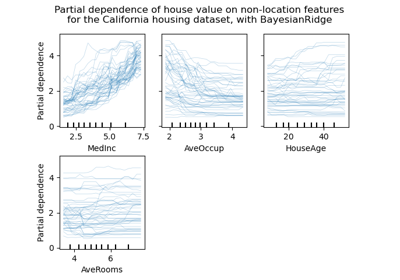Release Highlights for scikit-learn 0.24

Release Highlights for scikit-learn 0.24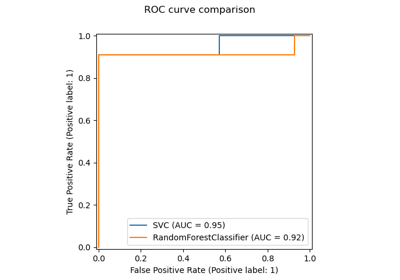Release Highlights for scikit-learn 0.22

Release Highlights for scikit-learn 0.22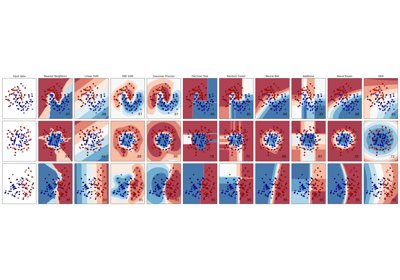Classifier comparison

Classifier comparison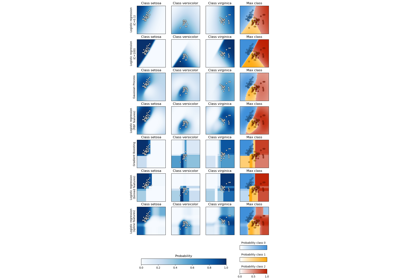Plot classification probability

Plot classification probabilityRecognizing hand-written digits

Recognizing hand-written digits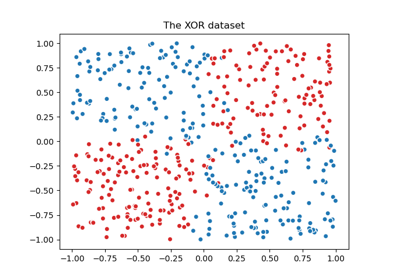Plot the decision boundaries of a VotingClassifier

Plot the decision boundaries of a VotingClassifier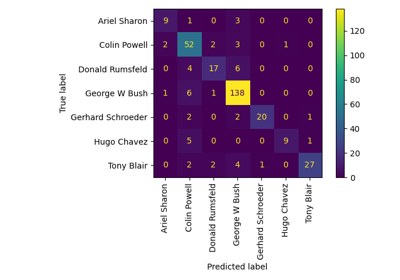Faces recognition example using eigenfaces and SVMs

Faces recognition example using eigenfaces and SVMsLibsvm GUI

Libsvm GUI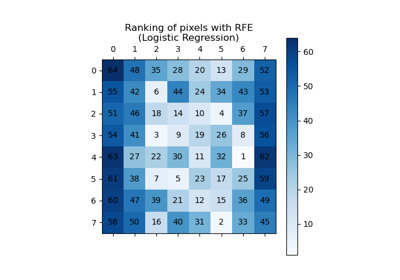Recursive feature elimination

Recursive feature elimination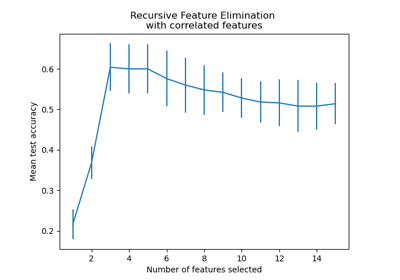Recursive feature elimination with cross-validation

Recursive feature elimination with cross-validation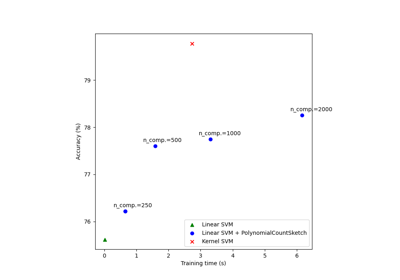Scalable learning with polynomial kernel approximation

Scalable learning with polynomial kernel approximationDisplaying Pipelines

Displaying Pipelines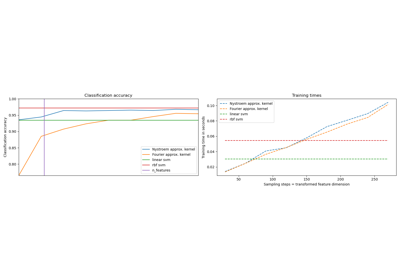Explicit feature map approximation for RBF kernels

Explicit feature map approximation for RBF kernels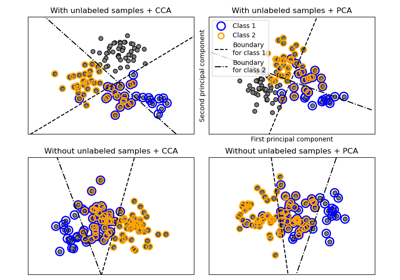Multilabel classification

Multilabel classification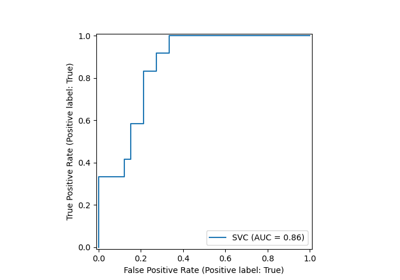ROC Curve with Visualization API

ROC Curve with Visualization API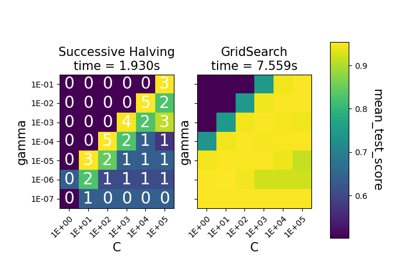Comparison between grid search and successive halving

Comparison between grid search and successive halving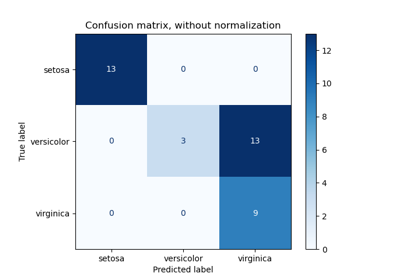Confusion matrix

Confusion matrixCustom refit strategy of a grid search with cross-validation

Custom refit strategy of a grid search with cross-validation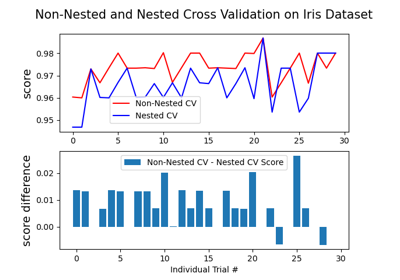Nested versus non-nested cross-validation

Nested versus non-nested cross-validation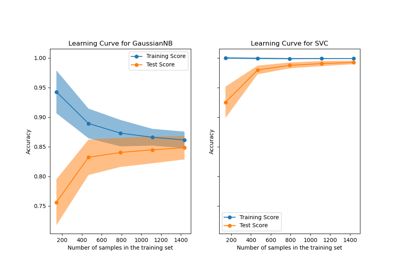Plotting Learning Curves

Plotting Learning CurvesPlotting Validation Curves

Plotting Validation Curves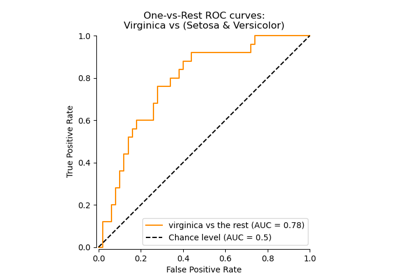Receiver Operating Characteristic (ROC)

Receiver Operating Characteristic (ROC)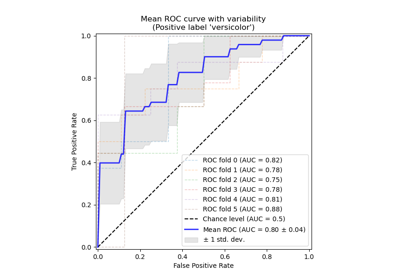Receiver Operating Characteristic (ROC) with cross validation

Receiver Operating Characteristic (ROC) with cross validation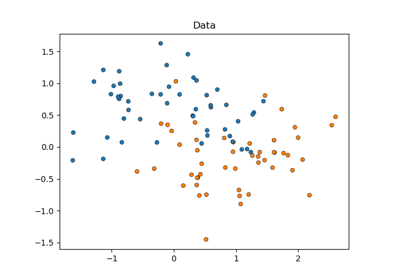Statistical comparison of models using grid search

Statistical comparison of models using grid search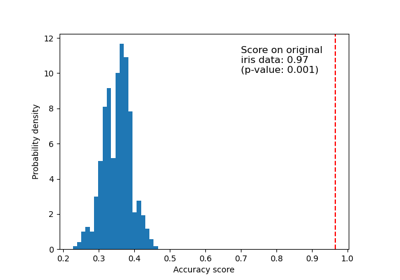Test with permutations the significance of a classification score

Test with permutations the significance of a classification scoreConcatenating multiple feature extraction methods

Concatenating multiple feature extraction methods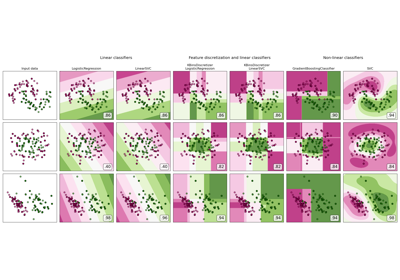Feature discretization

Feature discretization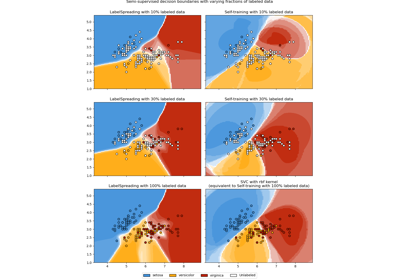Decision boundary of semi-supervised classifiers versus SVM on the Iris dataset

Decision boundary of semi-supervised classifiers versus SVM on the Iris dataset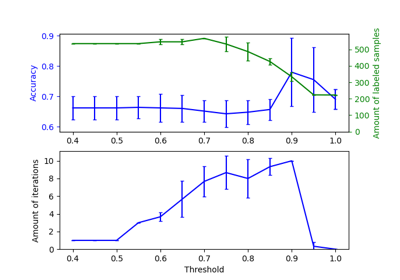Effect of varying threshold for self-training

Effect of varying threshold for self-training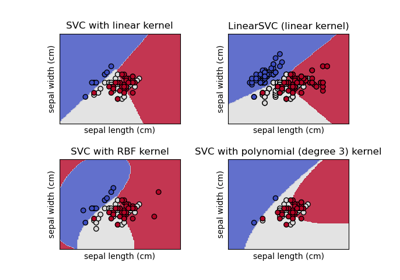Plot different SVM classifiers in the iris dataset

Plot different SVM classifiers in the iris dataset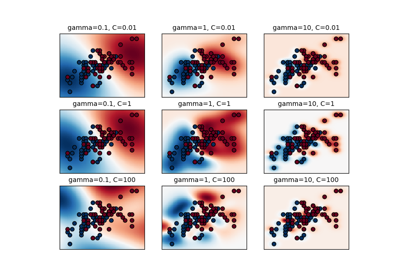RBF SVM parameters

RBF SVM parameters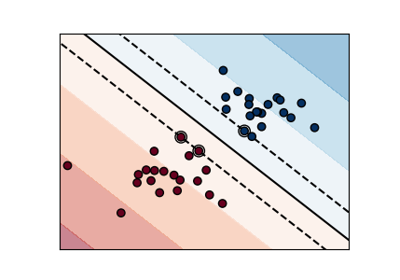SVM Margins Example

SVM Margins Example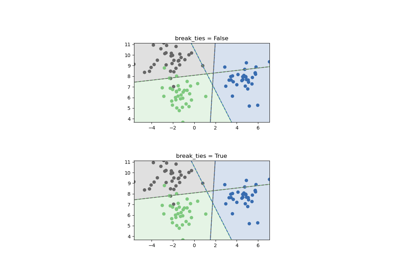SVM Tie Breaking Example

SVM Tie Breaking Example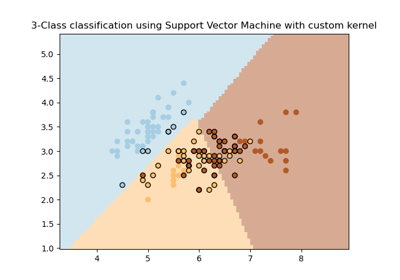SVM with custom kernel

SVM with custom kernel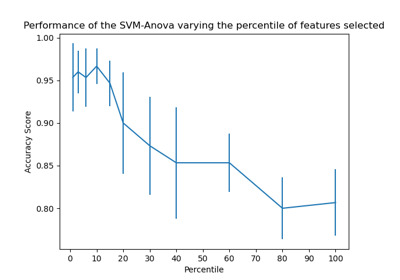SVM-Anova: SVM with univariate feature selection

SVM-Anova: SVM with univariate feature selection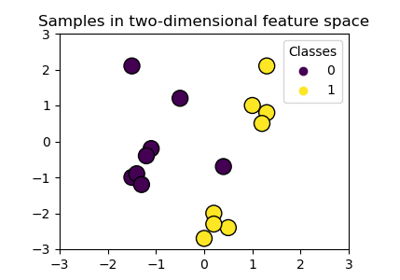SVM-Kernels

SVM-Kernels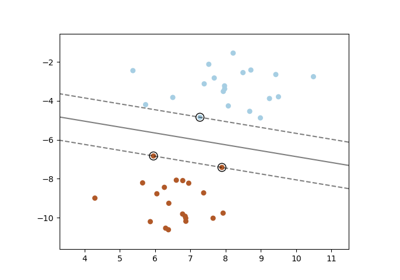SVM: Maximum margin separating hyperplane

SVM: Maximum margin separating hyperplane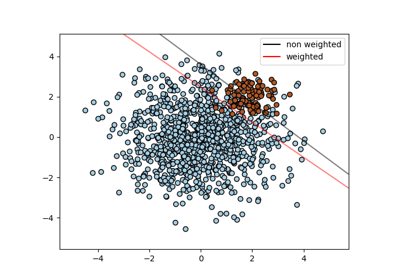SVM: Separating hyperplane for unbalanced classes

SVM: Separating hyperplane for unbalanced classes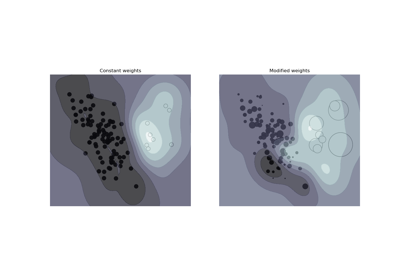SVM: Weighted samples

SVM: Weighted samplesCross-validation on Digits Dataset Exercise

Cross-validation on Digits Dataset ExerciseSVM Exercise

SVM Exercise